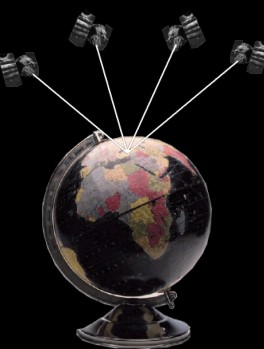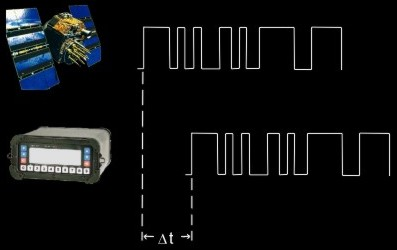How does GPS find positions?

• The receiver calculates a 3D position by measuring the ranges to at least 4 satellites.Figure 4.1: Positioning

• For the range measurement the receiver needs the two carriers containing code:

• C/A-Code with a lenght of 1 millisecond

• P-Code with a lenght of 7 days per satellite

• The receiver generates the same code as the satellite and calculates the time it took to receive the signal by synchronizing the signals.Figure 4.2: Determination of the time difference Dt

• The range to the satellite can be calculated using the time difference:

Range = Time Difference x Speed of Light

Auhtor: Michael Schulz, GEOSOFT